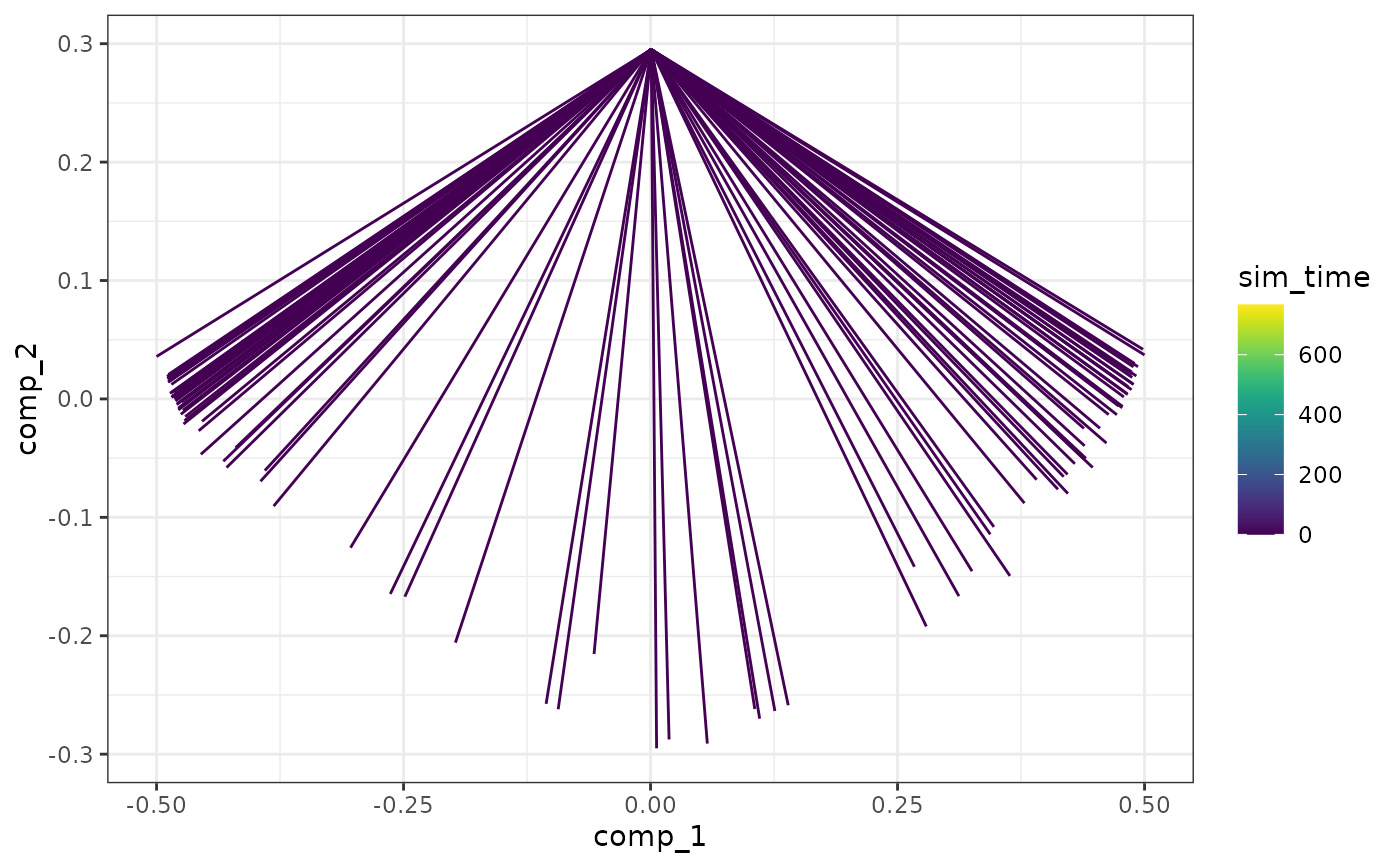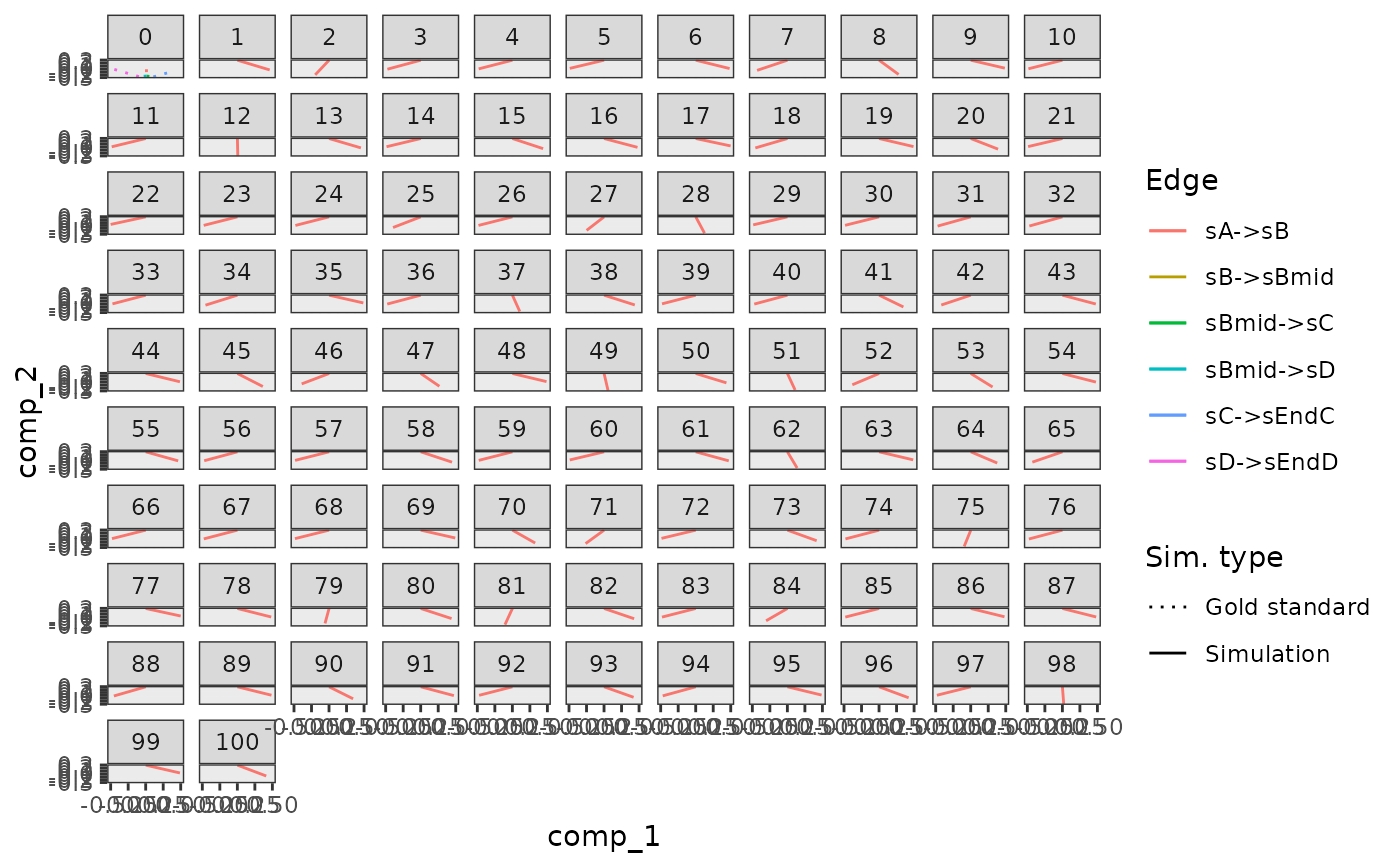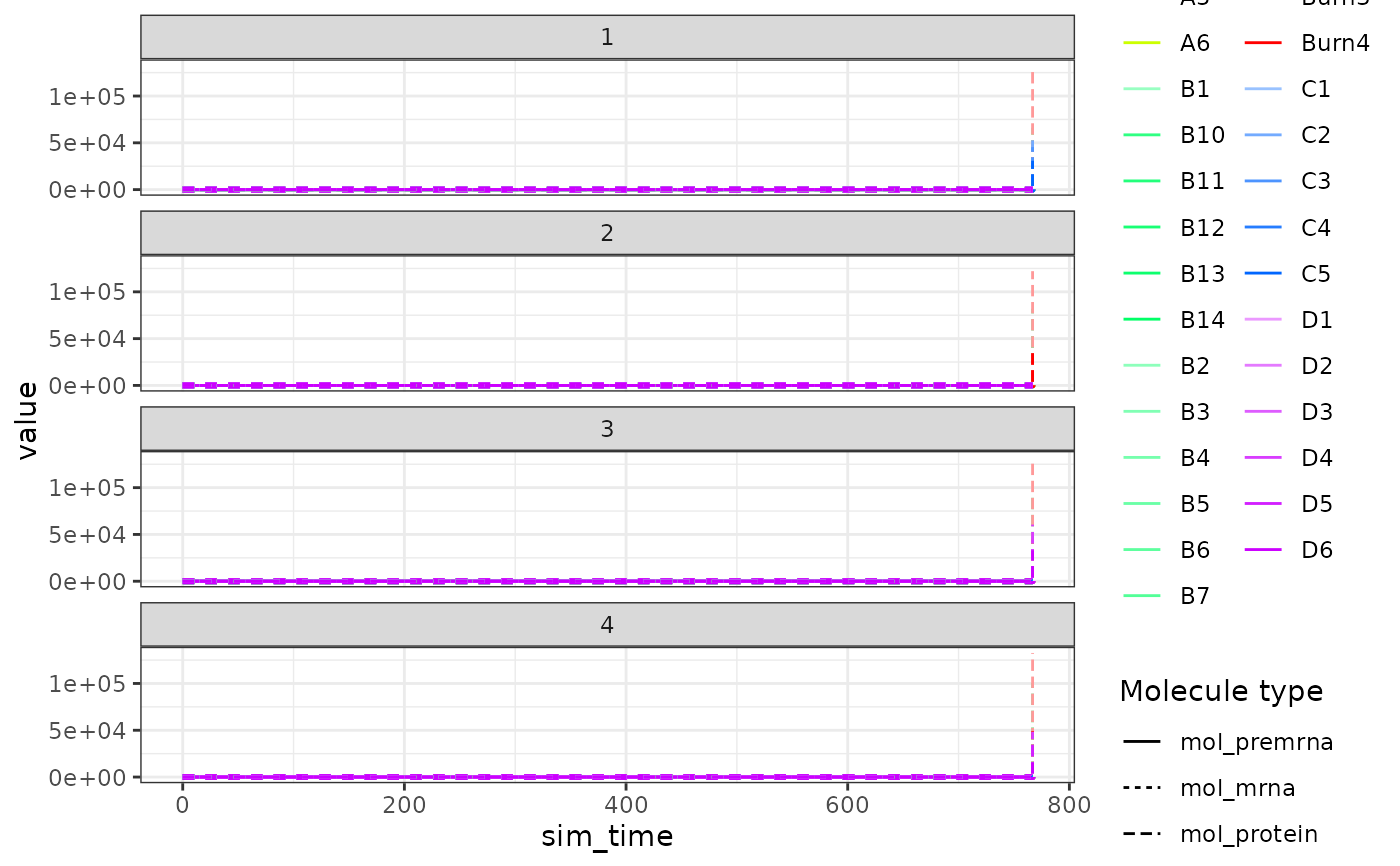generate_cells() runs simulations in order to determine the gold standard of the simulations. simulation_default() is used to configure parameters pertaining this process.

generate_cells(model)

simulation_default(
burn_time = NULL,
total_time = NULL,
ssa_algorithm = ssa_etl(tau = 30/3600),
census_interval = 4,
experiment_params = bind_rows(simulation_type_wild_type(num_simulations = 32),
simulation_type_knockdown(num_simulations = 0)),
store_reaction_firings = FALSE,
store_reaction_propensities = FALSE,
compute_cellwise_grn = FALSE,
compute_dimred = TRUE,
compute_rna_velocity = FALSE,
kinetics_noise_function = kinetics_noise_simple(mean = 1, sd = 0.005)
)

simulation_type_wild_type(
num_simulations,
seed = sample.int(10 * num_simulations, num_simulations)
)

simulation_type_knockdown(
num_simulations,
timepoint = runif(num_simulations),
genes = "*",
num_genes = sample(1:5, num_simulations, replace = TRUE, prob = 0.25^(1:5)),
multiplier = runif(num_simulations, 0, 1),
seed = sample.int(10 * num_simulations, num_simulations)
)

## Arguments

model

A dyngen intermediary model for which the gold standard been generated with generate_gold_standard().

burn_time

The burn in time of the system, used to determine an initial state vector. If NULL, the burn time will be inferred from the backbone.

total_time

The total simulation time of the system. If NULL, the simulation time will be inferred from the backbone.

ssa_algorithm

Which SSA algorithm to use for simulating the cells with GillespieSSA2::ssa()

census_interval

A granularity parameter for the outputted simulation.

experiment_params

A tibble generated by rbinding multiple calls of simulation_type_wild_type() and simulation_type_knockdown().

store_reaction_firings

Whether or not to store the number of reaction firings.

store_reaction_propensities

Whether or not to store the propensity values of the reactions.

compute_cellwise_grn

Whether or not to compute the cellwise GRN activation values.

compute_dimred

Whether to perform a dimensionality reduction after simulation.

compute_rna_velocity

Whether or not to compute the propensity ratios after simulation.

kinetics_noise_function

A function that will generate noise to the kinetics of each simulation. It takes the feature_info and feature_network as input parameters, modifies them, and returns them as a list. See kinetics_noise_none() and kinetics_noise_simple().

num_simulations

The number of simulations to run.

seed

A set of seeds for each of the simulations.

timepoint

The relative time point of the knockdown

genes

Which genes to sample from. "*" for all genes.

num_genes

The number of genes to knockdown.

multiplier

The strength of the knockdown. Use 0 for a full knockout, 0<x<1 for a knockdown, and >1 for an overexpression.

## Value

A dyngen model.

dyngen on how to run a complete dyngen simulation

## Examples

library(dplyr)
#>
#> Attaching package: ‘dplyr’
#> The following objects are masked from ‘package:stats’:
#>
#>     filter, lag
#> The following objects are masked from ‘package:base’:
#>
#>     intersect, setdiff, setequal, union
model <-
initialise_model(
backbone = backbone_bifurcating(),
simulation = simulation_default(
ssa_algorithm = ssa_etl(tau = .1),
experiment_params = bind_rows(
simulation_type_wild_type(num_simulations = 4),
simulation_type_knockdown(num_simulations = 4)
)
)
)
# \donttest{
data("example_model")
model <- example_model %>% generate_cells()
#> Warning: Simulation does not contain all gold standard edges. This simulation likely suffers from bad kinetics; choose a different seed and rerun.

plot_simulations(model)plot_gold_mappings(model)plot_simulation_expression(model)# }